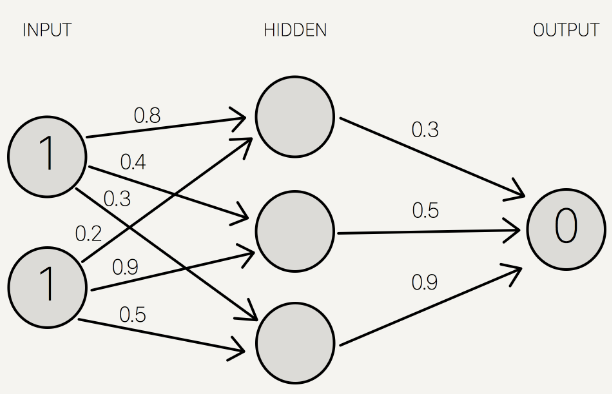2 views

Say for my first input layer I have 10 input nodes/neurons. Say my hidden layer has 10 neurons as well. My third and final layer is one output neuron.

How do I connect the layers? Is there a technique for determining the best way to do this or do you just connect each input neuron to each of the hidden layer neurons for a total of 100 edges between the two layers?

This is probably a really basic question but I haven't seen too many concrete examples. The examples I did find either seem to connect all the neurons or the connections seem randomized.

by (108k points)

I will explain with the help of an example;

See the bellow diagram:In the diagram, there are 2 input nodes and 3 hidden nodes and only one output node.

The connections between the nodes are not made randomly.

There is a way of doing this. Firstly we will connect the first node of the input layer to all the nodes of the adjacent hidden layer. The second node of the input layer follows the same technique as the first one. Then from the hidden layer, only one connection is established between the hidden node and the output node. One node-one connection follows here.

As you can see in the diagram that there are 3 hidden nodes and the connections are also 3.

So, according to your question, there are 10 input nodes,10 hidden nodes, and one output node then there must be 110 connections as 10 input nodes should connect to all 10 nodes in the hidden layer (100 connections). Then all 10 nodes in the hidden layer should connect to the output node (10 connections).

Hope this helps!

If you wish to know about Neural Network then visit this Neural Network Tutorial.

+1 vote